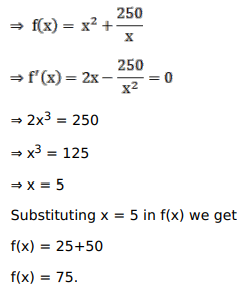# Solve this following

Question:

Mark $(\sqrt{ })$ against the correct answer in the following:

The minimum value of $\left(\mathrm{x}^{2}+\frac{250}{\mathrm{x}}\right)$ is

A. 0

B. 25

C. 50

D. 75

Solution: